Shipra Saxena — March 17, 2021

## Objective

• In sequence modeling techniques, the Gated Recurrent Unit is the newest entrant after RNN and LSTM, hence it offers an improvement over the other two.
• Understand the working of GRU and how it is different from LSTM

## Introduction

GRU or Gated recurrent unit is an advancement of the standard RNN i.e recurrent neural network. It was introduced by Kyunghyun Cho et al in the year 2014.

Note: If you are more interested in learning concepts in an Audio-Visual format, We have this entire article explained in the video below. If not, you may continue reading.

GRUs are very similar to Long Short Term Memory(LSTM). Just like LSTM, GRU uses gates to control the flow of information. They are relatively new as compared to LSTM. This is the reason they offer some improvement over LSTM and have simpler architecture.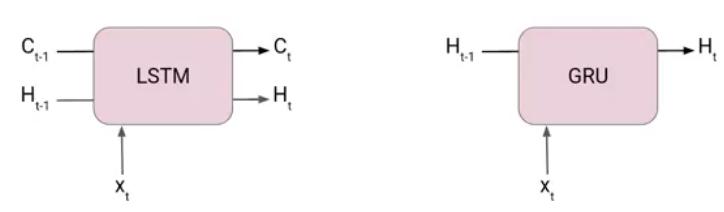Another Interesting thing about  GRU is that, unlike LSTM, it does not have a separate cell state (Ct). It only has a hidden state(Ht). Due to the simpler architecture, GRUs are faster to train.

In case you are unaware of the LSTM network, I will suggest you go through the following article-

## The architecture of Gated Recurrent Unit

Now lets’ understand how GRU works. Here we have a GRU cell which more or less similar to an LSTM cell or RNN cell.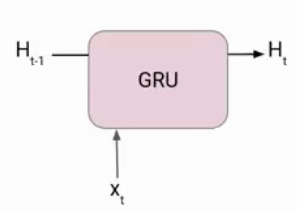At each timestamp t, it takes an input Xt and the hidden state Ht-1 from the previous timestamp t-1. Later it outputs a new hidden state Ht which again passed to the next timestamp.

Now there are primarily two gates in a GRU as opposed to three gates in an LSTM cell. The first gate is the Reset gate and the other one is the update gate.

### Reset Gate (Short term memory)

The Reset Gate is responsible for the short-term memory of the network i.e the hidden state (Ht). Here is the equation of the Reset gate.If you remember from the LSTM gate equation it is very similar to that. The value of rt will range from 0 to 1 because of the sigmoid function. Here Ur and Wr are weight matrices for the reset gate.

### Update Gate (Long Term memory)

Similarly, we have an Update gate for long-term memory and the equation of the gate is shown below.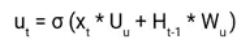The only difference is of weight metrics i.e Uu and Wu.

## How GRU Works

Now let’s see the functioning of these gates. To find the Hidden state Ht in GRU, it follows a two-step process. The first step is to generate what is known as the candidate hidden state. As shown below

### Candidate Hidden State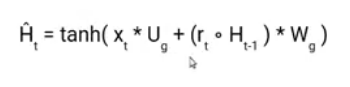It takes in the input and the hidden state from the previous timestamp t-1 which is multiplied by the reset gate output rt. Later passed this entire information to the tanh function, the resultant value is the candidate’s hidden state.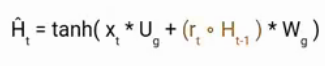The most important part of this equation is how we are using the value of the reset gate to control how much influence the previous hidden state can have on the candidate state.

If the value of rt is equal to 1 then it means the entire information from the previous hidden state Ht-1 is being considered. Likewise, if the value of rt is 0 then that means the information from the previous hidden state is completely ignored.

### Hidden state

Once we have the candidate state, it is used to generate the current hidden state Ht. It is where the Update gate comes into the picture. Now, this is a very interesting equation, instead of using a separate gate like in LSTM in GRU we use a single update gate to control both the historical information which is Ht-1 as well as the new information which comes from the candidate state.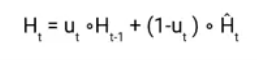Now assume the value of ut is around 0 then the first term in the equation will vanish which means the new hidden state will not have much information from the previous hidden state. On the other hand, the second part becomes almost one that essentially means the hidden state at the current timestamp will consist of the information from the candidate state only.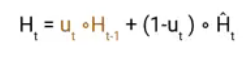Similarly, if the value of ut is on the second term will become entirely 0 and the current hidden state will entirely depend on the first term i.e the information from the hidden state at the previous timestamp t-1.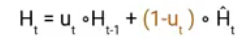Hence we can conclude that the value of ut is very critical in this equation and it can range from 0 to 1.

In case, you are interested to know more about GRU I suggest you read this Paper.

## End Notes

So just to summarize, Let’s see how different GRU is from LSTM.

LSTM has three gates on the other hand GRU has only two gates. In LSTM they are the Input gate, Forget gate, and Output gate. Whereas in GRU we have a Reset gate and Update gate.

In LSTM we have two states Cell state or Long term memory and Hidden state also known as Short term memory. In the case of GRU, there is only one state i.e Hidden state (Ht).

If you are looking to kick start your Data Science Journey and want every topic under one roof, your search stops here. Check out Analytics Vidhya’s Certified AI & ML BlackBelt Plus Program